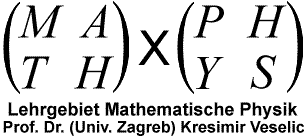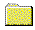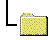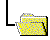Lehrgebiet Mathematische PhysikMitarbeiterProf. Dr. (Univ. Zagreb) K. VeselićSchriftenverzeichnis / List of Papers

## Schriftenverzeichnis / List of Papers

1. S. Kurepa, K. Veselić, Transformation of a matrix to normal forms, Glasnik Mat. 2 (22), (1967) 38-51.
2. K. Veselić, On spectral concentration for some classes of selfadjoint operators, Glasnik Mat. 4 (24), (1969) 213-229.
3. K. Veselić, The nonrelativistic limit of the Dirac equation and the spectral concentration, Glasnik Mat. 4 (24), (1969) 231-241.
4. K. Veselić, A perturbation theorem for a class of J-selfadjoint operators, Institut `Rudjer Boskovic' preprint IRB-TP-6-69.
5. K. Veselić, A spectral theory for the Klein-Gordon equation with an external electrostatic potential, Nucl. Phys. 147, (1970) 215-224.
6. K. Veselić, A spectral theorem for a class of J-normal operators, Glasnik Mat. 5 (25), (1970) 97-102.
7. K. Veselić, On perturbation theory for a class of J-selfadjoint operators, Glasnik Mat. 5 (25), (1970) 103-108.
8. K. Veselić, Perturbation of pseudoresolvents and analyticity in 1/c in relativistic Quantum Mechanics, Communs. Math. Phys. 22, (1971) 27-43.
9. D. Miličić, K. Veselić, On the boundary of essential spectra, Glasnik Mat. 6 (26), (1971) 73-78.
10. K. Veselić, On spectral concentration for a class of J-selfadjoint operators, Glasnik Mat. 6 (26), (1971) 285-299.
11. K. Veselić, On spectral properties of a class of J-selfadjoint operators I, Glasnik Mat. 7 (27), (1972) 229-248.
12. K. Veselić, On spectral properties of a class of J-selfadjoint operators II, Glasnik Mat. 7 (27), (1972) 249-254.
13. K. Veselić, J. Weidmann, Existenz der Wellenoperatoren für eine allgemeine Klasse der Operatoren, Math. Z. 134, (1973) 255-274.
14. K. Veselić, J. Weidmann, Scattering theory for a general class of differential operators, Proceedings of the conference on Spectral Theory and asymptotics of differential equations, Scheveningen 1973.
15. K. Veselić, On the Jordan form in a unitary space, Lin. Algebra Appl. 8, (1974) 507-514.
16. K. Veselić, J. Weidmann, Asymptotic estimates of wave functions and the existence of the wave operators, J. Funct. Anal. 17, (1974) 61-77.
17. K. Veselić, On the Jordan form in a unitary space II, Glasnik Mat. 10 (30), (1975) 35-42.
18. K. Veselić, A convergent Jacobi method for solving the eigenproblem of arbitrary real matrices, Numer. Math. 25, (1976) 179-184.
19. K. Veselić, On essential spectra in Banach algebras, Glasnik Mat. 10 (30), (1975) 295-310.
20. K. Veselić, Some convergent Jacobi-like procedures for diagonalising J-symmetric matrices, Numer. Math. 27, (1976) 67-75.
21. H. Kraljević, K. Veselić, On algebraic and spectrally finite Banach algebras, Glasnik Mat. 11 (31), (1976) 291-318.
22. K. Veselić, J. Weidmann, Potential scattering in a homogeneous electrostatic field , Math. Z. 156, (1977) 93-104.
23. K. Veselić, On a class of Jacobi-like procedures for diagonalising arbitrary real matrices, Numer. Math. 33, (1979) 157-172.
24. K. Veselić, On a new class of elementary matrices, Numer. Math. 33, (1979) 173-180.
25. I. Aganović K. Veselić, On a singular contact problem for two dimensional Laplace equation, Rendiconti di Matem. 11, (1979) 521-532.
26. K. Veselić, Mathematische Methoden der Theoretischen Mechanik, Vorlesungsskriptum, Universität Dortmund 1978.
27. K. Veselić, On real eigenvalues of real tridiagonal matrices, Linear Algebra Appl. 27, (1979) 167-171.
28. K. Veselić, H. J. Wenzel, A quadratically convergent Jacobi-like method for real matrices with complex eigenvalues, Numer. Math. 33, (1979) 425-435.
29. K. Veselić, On optimal linearisations of a quadratic eigenvalue problem, Linear and Multilinear Algebra 8, (1980) 253-258.
30. D. Meyer, K. Veselić, On some new inclusion theorems for the eigenvalues of partitioned matrices, Numer. Math. 34, (1980) 431-437.
31. A. Erdmann, K. Veselić, A new norm-reducing algorithm for diagonalizing arbitrary matrices, Fernuniversität Hagen preprint 1981.
32. H. Kraljević, S. Suljagić, K. Veselić, Index in semisimple Banach algebras, Glasnik Mat. 17 (37), (1982) 73-95.
33. K. Veselić, On the non-relativistic limit of the bound states of the Klein-Gordon equation, J. Math. Anal. Appl. 96, (1983) 63-84.
34. K. Veselić, A global Jacobi method for a symetric indefinite problem S x = lambda T x , Comp. Methods Appl. Mech. Engrg. 38, (1983) 273-290.
35. V. Enss, K. Veselić, Bound states and propagating states for time-dependent Hamiltonians, Ann. Inst. Henri Poincare A XXXIX, (1983) 159-191.
36. K. Veselić, Constructive Linear Algebra, Buchmanuskript, 1983.
37. P. Hoppe, K. Veselić, A Jacobi-like method for the symmetric indefinite eigenproblem S x = lambda T x with complex eigenvalues, Glasnik Mat. 18 (38), (1983) 383-390.
38. R. Danisch, K. Delinić, K. Veselić, Quadratic eigenvalue solver for modal response analysis of non-proportionally damped elastic structures, Trans. 7-th Int. Conf. on Struct. Mech. in Reactor Technology, Chicago 1983, K 3/1 North-Holland 1983.
39. K. Veselić, Mathematische Methoden der Physik und Technik I, Fernkurs, Fernuniversität Hagen, 1984.
40. I. Aganović, K. Veselić, Gleichungen der Mathematischen Physik I (Serbokroatisch) Skolska Knjiga Zagreb 1985.
41. K. Veselić, Warum müssen Ingenieure Mathematik lernen?, Gesellschaft der Freunde der Fernuniversität, Jahrbuch, Hagen 1986.
42. K. Veselić, De forma catenarum in campo gravitatis pendentium, in Klasična Gimnazija u Zagrebu (1607-1987) Latina et Graeca Zagreb 1987 pp. 115-117.
43. V. Hari, K. Veselić, On Jacobi methods for singular value decompositions, SIAM J. Sci. Stat. Comput. 8, (1987) 741-754.
44. I. Aganović, K. Veselić, Einführung in die partiellen Differentialgleichungen, Fernuniversität Hagen Fernkurs, 1987.
45. K. Veselić, On linear vibrational systems with one dimensional damping, Appl. Anal. 29 (1988) 1-18.
46. K. Veselić, V. Hari, A note on a one-sided Jacobi algorithm, Numer. Math. 56 (1989) 627 - 633.
47. I. Aganović, K. Veselić, Einführung in die partiellen Differentialgleichungen II, Fernuniversität Hagen Fernkurs, ...
48. K. Veselić, On linear vibrational systems with one dimensional damping II, Integral Eq. Operator Th. 13 (1990) 883-897.
49. K. Veselić, Optimising the solution of a Ljapunov equation, Fernuniversität Hagen preprint 1990.
50. I. Aganović, K. Veselić, Einführung in die Analytische Mechanik, Mat. Odj. PMF, Zagreb 1990 (serbokroatisch).
51. A. Mikelić, A. Suhadolc, K. Veselić, On the potential flow of an ideal incompressible fluid through a porous boundary, IMA J. Appl. Math. 47 109 - 125 (1991).
52. K. Veselić, A spectral theory of the Klein-Gordon equation involving a homogeneous electric field, J. Operator Th. 25 (1991) 319-330.
53. M. Kovač-Striko, K. Veselić, Some remarks on the spectra of hermitean matrices, Linear Algebra Appl. 145 (1991) 221 - 229.
54. H. Langer, B. Najman, K. Veselić, Perturbation of the eigenvalues of quadratic matrix polynomials, SIAM J. Matrix Anal. Appl. 13 (1992) 474-489.
55. I. Aganović K. Veselić, Lineare Differentialgleichungen (kroatisch) Matem. Odjel PMF Sveucilista u Zagrebu Zagreb 1992.
56. J. Demmel, K. Veselić, Jacobi's method is more accurate than QR, SIAM J. Matrix Anal. Appl. 13 (1992) 1204-1245.
57. K. Veselić, I. Slapničar, Floating-point perturbations of Hermitian matrices, Linear Algebra Appl 195 (1993) 81 - 116.
58. K. Veselić, A Jacobi eigenreduction algorithm for definite matrix pairs, Numer. Math. 64 (1993) 241-269.
59. K. Veselić, On rank-one perturbations of definite matrix pairs, Fernuniversität Hagen preprint 1993.
60. Z. Drmač, M. Omladič, K. Veselić, On the perturbation of the Cholesky factorisation, SIAM J. Matrix Anal. Appl. 15 (1994) 1319 - 1332.
61. K. Veselić, On the stability of rotating systems, ZAMM 75 (1994)4 325 - 328.
62. I. Slapničar, K. Veselić, Perturbations of the eigenprojections of a factorised Hermitian matrix, Linear Algebra Appl. 218 (1995) 273-280.
63. K. Veselić, Finite catenary and the method of Lagrange, SIAM R., 37 (1995) 224-229.
64. M. Kovač-Striko, K. Veselić, Trace minimization and definiteness of symmetric matrix pairs, Linear Algebra Appl. 216 (1995) 139-158.
65. Z. Drmač, K. Veselić, Note on the accuracy of the eigensolution of matrices generated by finite elements, SVD AND SIGNAL PROCESSING, III (M. Moonen and B. de Moor Eds.) Elsevier 1995, pp. 115-221.
66. K. Veselić, De forma catenarum in campo gravitatis pendentium, Vox Latina 31 (1995) 237-242.
67. R. Mathias and K. Veselić A relative perturbation bound for eigenvectors, Linear Algebra Appl . 270 (1998) 315-321.
68. K. Veselić, Note on the accuracy of symmetric eigenreduction algorithms, ETNA 4 (1996) 37-45.
69. B. Najman, K. Veselić, Multiplicative perturbations of positive operators in Krein spaces, Operator Theory: Advances and Applications 106 (1998) 328-336.
70. K. Veselić, Exponential decay of semigroups in Hilbert space, Semigroup Forum 55 (1997) 99-109.
71. I. Aganović, Z. Tutek, K. Veselić, Decoupling of a system of two membranes by a thin rigid cylinder, ZAMM 77 (1997) S507-508
72. M. Kovač-Striko, K. Veselić, Block Diagonalization of nearly diagonal matrices, Glasnik Mat. 36(56) (2001) 271-286.
73. I. Slapničar, K. Veselić, A bound for the condition of hyperbolic eigenvector matrix, Linear Algebra Appl. 290 (1999) 247-255.
74. K. Veselić, Estimating the operator exponential, Linear Algebra Appl. 280 (1998) 241-244.
75. Z. Drmač, K. Veselić, On the singular value decomposition of matrices generated by finite elements, Fernuniversität Hagen preprint 1995.
76. J. Demmel, M. Gu, S. Eisenstat, I. Slapničar, K. Veselić, Z. Drmač, Computing the singular value decomposition with high relative acuracy, Linear Algebra Appl. 299 (1999) 12-80.
77. K. Veselić, A note on the pivoted symmetric LR iteration, Numer. Math. 83 (1999) 699-702.
78. K. Veselić, Visualized optimization in Mathematical Physics (a classroom consideration), Proc. 7. Int. Conf. Operational Res. Croatian Operational Research Society (1999) 3-9.
79. K. Veselić, K. Brabender, K. Delinić, Passive control of linear systems, in Applied Mathematics and Computation, M. Rogina et al. Eds. Dept. of Math. Univ. Zagreb, Zagreb 2001, pp. 39-68.
80. I. Aganović, Z. Tutek, K. Veselić, Approximation of Green's function and application, Glasnik Mat. 35(55) (2000) 179-190.
81. K. Veselić, I. Slapničar, On spectral condition of J-Hermitian operators, Glasnik Mat. 35(55) (2000) 3-23.
82. Z. Drmač, K. Veselić, Approximate eigenvectors as preconditioner, Linear Algebra Appl. 309 (2000) 191-215.
83. K. Veselić, Perturbation theory for the eigenvalues of factorised symmetric matrices, Linear Algebra Appl. 309 (2000) 85-102.
84. K. Veselić, Bounds for exponentially stable semigroups, Linear Algebra Appl. 358 (2003) 309-333.
85. I. Nakić, K. Veselić, Wielandt and Ky-Fan theorem for matrix pairs, Linear Algebra Appl. 369 (2003) 77-93.
86. S. Cox, I. Nakić, A. Rittmann, K. Veselić, Lyapunov optimization of a damped system, Systems & Control Letters, 53 (2004) 187-194.
87. N. Truhar, K. Veselić, Optimizing the solution of the Lyapunov equation, Fernuniversität Hagen preprint 2001.
88. K. Veselić, On the convergence of the Newton iteration, ZAMM 84 (2004) 147-157.
89. L. Grubišić, K. Veselić, On Ritz Approximations for Positive Definite Operators I (Theory), Linear Algebra Appl. 417 (2006) 397-422.
90. Z. Drmač, V. Hari, L. Sopta, Z. Tutek, K. Veselić (Eds.), Applied Mathematics and Scientific Computing (Proc. II Conf. Appl. Math. Sci. Comp. Dubrovnik 2001), Kluwer 2003.
91. K. Veselić, Energy Decay of Damped Systems, ZAMM, 84 (2004) 856-864.
92. N. Truhar, K. Veselić, On some properties of the Lyapunov equation for damped systems, Math. Commun. 9, No.2, (2004) 189-197.
93. K. Veselić, Bounds for contractive semigroups and second order systems, Operator Theory: Advances and Applications, 162, (2005) 293-308.
94. Z. Drmač, K. Veselić, New fast and accurate Jacobi algorithm I, SIMAX 299 (2008) 1322-1342.
95. Z. Drmač, K. Veselić, New fast and accurate Jacobi algorithm II, SIMAX 30 (2008) 1343-1362.
96. L. Grubišić, K. Veselić, On weakly formulated Sylvester equations and applications, Integral Eq. Operator Th. 58 (2007) 175-204.
97. N. Truhar, K. Veselić, Bounds on the trace of a solution to the Lyapunov equation with a general stable matrix, Systems & Control Letters 56 (2007) 493-503.
98. N. Truhar, K. Veselić, An efficient method for estimating the optimal dampers' viscosity for linear vibrational systems using Lyapunov equation, SIMAX 31 (2009) 18--39.
99. K. Veselić, Spectral perturbation bounds for selfadjoint operators I, Operators and Matrices, 2 (2008) 307-340.
100. K. Veselić and N. Truhar, On bounds for discrete semigroups, Operators and Matrices. 3 (2009) , 3; 357-365
101. K. Veselić, Note on interlacing for hyperbolic quadratic pencils, Operator Theory: Advances and Applications 198 (2009) 305--307.
102. L. Grubišić, Vadim Kostrykin, Konstantin A. Makarov and K. Veselić, 2 x 2 matrices, quadratic forms, and representation theorems (Oberwolfach Reports, Volume 6, Issue 4, 2009, pp. 2988 - 2989. published by European Mathematical Society) A preprint is available from the MFO webpage.
103. L. Grubišić, V. Kostrykin, K. A. Makarov. K. Veselić, Representation Theorems for Indefinite Quadratic Forms Revisited, Mathematika, Volume 59, Issue 01, pp. 169-189 (2013). A preprint is available from ArXiv.org.
104. L. Grubišić, V. Kostrykin, K. A. Makarov. K. Veselić, The TAN 2 Theta Theorem for Indefinite Quadratic Forms , Journal of Spectral Theory, Volume 3, Issue 1 (2013), pp. 83-100 (A preprint is available from ArXiv.org).
105. Z. Tomljanović, N. Truhar and K. Veselić, Optimizing a damped system - a case study, International Journal of computer mathematics, 88 (2011) , 7; 1533-1545.
106. K. Veselić, Damped Oscillations of Linear Systems --- a mathematical introduction, Springer Lecture Notes in Mathematics 2023, (2011)
107. L. Grubišić, N. Truhar, K. Veselić, The Rotation of Eigenspaces of Perturbed Matrix Pairs. Linear algebra and its applications, 436, (2012) 4161-4178.
108. K. Veselić, Catenary --- an elementary and complete approach (Slovenian), Obzornik za matematiko in fiziko 59 (6) (2012) 201-204.
109. I. Veselić, K. Veselić, Spectral gap estimates for some block matrices, Operators and Matrices 9 (2015) 241-275.
110. N. Truhar, Z. Tomljanović, K. Veselić, Damping optimization in mechanical systems with external force, Applied Mathematics and Computation 250, 1 (2015) 270-279.
111. I. Aganović, K, Veselić, Matematičke metode i modeli (Mathematical Methods and Models, Croatian) 432 p. Sveučilište Josip Juraj Strossmayer u Osijeku -- Odjel za matematiku 2014.
112. L. Grubišić, Vadim Kostrykin, Konstantin A. Makarov and K. Veselić, The Tan 2Θ-theorem in fluid dynamics, arXiv:1708.00509, J. Spectr. Theory 9, 4 (2019) 1431-1457 DOI: 10.4171/JST/282
113. I. Nakić, K. Veselić, Perturbation of eigenvalues of the Klein-Gordon operators, arXiv:1807.11612, Revista Matemática Complutense, 33, 557-581 (2020)
114. A. Seelmann, M. Täufer, K. Veselić, Protecting points from operator pencils, arXiv:1908.08897, to appear in J. Operator Th. (2020)
115. Z. Drmač, K. Veselić, Note on the accuracy of the eigensolution of matrices generated by finite elements, SVD AND SIGNAL PROCESSING, III (M. Moonen and B. de Moor Eds.) Elsevier 1995, pp. 115-221.
K.V.